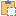#### Opening Class

An opening in an Autodesk Revit project or family document.

Namespace: Autodesk.Revit.DB
Assembly: RevitAPI (in RevitAPI.dll) Version: 2015.0.0.0 (2015.0.0.0)

# Syntax

C#
``public class Opening : Element``
Visual Basic
``````Public Class Opening _
Inherits Element``````
Visual C++
``public ref class Opening : public Element``

# Remarks

The object represents a variety of different types of openings:
• A rectangular opening in a wall created by two boundary points in a revit project.
• An opening created by a set of curves applied to a roof, floor, ceiling, beam, brace or column.
• A vertical shaft opening extending one or more levels.
• A simple opening created on a wall or ceiling in a family document.
Depending upon the type of opening, some of the properties of this class will not be available.

# Remarks

This object derived from the Element base object and such supports all the methods of that object such as the ability to retrieve the parameters of that object. This object also supports access to a structural analytical model but this feature is only available with Autodesk Revit Structure.

# ExamplesCopy C#
``````private void Getinfo_Opening(Opening opening)
{
string message = "Opening:";

//get the host element of this opening
message += "\nThe id of the opening's host element is : " + opening.Host.Id.IntegerValue;

//get the information whether the opening has a rect boundary
//If the opening has a rect boundary, we can get the geometry information from BoundaryRect property.
//Otherwise we should get the geometry information from BoundaryCurves property
if (opening.IsRectBoundary)
{
message += "\nThe opening has a rectangular boundary.";
//array contains two XYZ objects: the max and min coords of boundary
IList<XYZ> boundaryRect = opening.BoundaryRect;

//get the coordinate value of the min coordinate point
XYZ point = opening.BoundaryRect;
message += "\nMin coordinate point:(" + point.X + ", "
+ point.Y + ", " + point.Z + ")";

//get the coordinate value of the Max coordinate point
point = opening.BoundaryRect;
message += "\nMax coordinate point: (" + point.X + ", "
+ point.Y + ", " + point.Z + ")";
}
else
{
message += "\nThe opening doesn't have a rectangular boundary.";
// Get curve number
int curves = opening.BoundaryCurves.Size;
message += "\nNumber of curves is : " + curves;
for (int i = 0; i < curves; i++)
{
Autodesk.Revit.DB.Curve curve = opening.BoundaryCurves.get_Item(i);
// Get curve start point
message += "\nCurve start point: " + XYZToString(curve.GetEndPoint(0));
// Get curve end point
message += "; Curve end point: " + XYZToString(curve.GetEndPoint(1));
}
}
}

// output the point's three coordinates
string XYZToString(XYZ point)
{
return "(" + point.X + ", " + point.Y + ", " + point.Z + ")";
}``````Copy VB.NET
``````Private Sub Getinfo_Opening(opening As Opening)
Dim message As String = "Opening:"

'get the host element of this opening
message += vbLf & "The id of the opening's host element is : " & Convert.ToString(opening.Host.Id.IntegerValue)

'get the information whether the opening has a rect boundary
'If the opening has a rect boundary, we can get the geometry information from BoundaryRect property.
'Otherwise we should get the geometry information from BoundaryCurves property
If opening.IsRectBoundary Then
message += vbLf & "The opening has a rectangular boundary."
'array contains two XYZ objects: the max and min coords of boundary
Dim boundaryRect As IList(Of XYZ) = opening.BoundaryRect

'get the coordinate value of the min coordinate point
Dim point As XYZ = opening.BoundaryRect(0)
message += ((vbLf & "Min coordinate point:(" + point.X & ", ") + point.Y & ", ") + point.Z & ")"

'get the coordinate value of the Max coordinate point
point = opening.BoundaryRect(1)
message += ((vbLf & "Max coordinate point: (" + point.X & ", ") + point.Y & ", ") + point.Z & ")"
Else
message += vbLf & "The opening doesn't have a rectangular boundary."
' Get curve number
Dim curves As Integer = opening.BoundaryCurves.Size
message += vbLf & "Number of curves is : " & curves
For i As Integer = 0 To curves - 1
Dim curve As Autodesk.Revit.DB.Curve = opening.BoundaryCurves.Item(i)
' Get curve start point
message += vbLf & "Curve start point: " & XYZToString(curve.GetEndPoint(0))
' Get curve end point
message += "; Curve end point: " & XYZToString(curve.GetEndPoint(1))
Next
End If